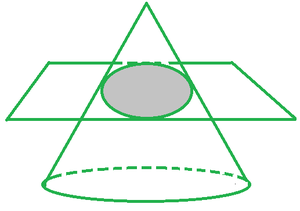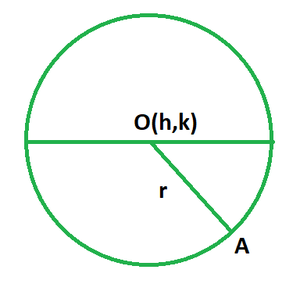GeeksforGeeks App
Open AppBrowser
Continue

# Complete the square and write the equation of the circle in standard form x2 + y2 + 10x – 10y +41 = 0

Conic sections also referred to as conics, are formed when a plane intersects a cone. The shape of such sections depends upon the angle at which the intersection happens. As such there are four types of conic sections, namely the circle, ellipse, parabola and hyperbola. Each of these shapes has its own properties and equations in mathematics. The circle is discussed below.

Circle

In mathematics, the circle can be studied as two different things: as a 2D shape in mensuration and as a conic section in 3D geometry. While its properties may remain the same, the calculations and algorithms pertaining to it are a bit different.

As a conic section, a circle is a shape formed when a plane intersects a cone perpendicular to its axis. In other words, a circle is formed when a plane cuts a right-angled cone at 90°.As shown in the figure above, a right-angled cone and a plane intersect at a right angle and the shaded region is the circle formed because of the intersection.

Equation of a Circle• The standard form of equation with Centre (h, k) and radius r is given as:

(x − h)2 + (y − k)2 = r2

• The general form of equation of a circle is:

x2 + y2 + 2gx + 2fy + c = 0

### Solution:

Complete the square for x2 − 10x.

(x + 5)2 − 25 + y2 − 10y = −41

⇒ (x + 5)2 + y2 − 10y = −41 + 25

Now complete the square for y2 − 10y.

⇒ (x + 5)2 + (y − 5)2 − 25 = −41 + 25

⇒ (x + 5)2 + (y − 5)2 = −41 + 25 + 25

⇒ (x + 5)2 + (y − 5)2 = 9

### Similar Problems

Problem 1: Find the standard equation of a circle with centre at (1,2) and radius 4.

Solution:

The standard form of equation with Centre (h, k) and radius r is given as:

(x − h)2 + (y − k)2 = r2

Given: The center is (3,4) and the radius is 5.

The equation of circle is:

(x−1)2 + (y−2)2 = 42

⇒ x2 +y2 − 2x + 1 − 4y + 4 = 25

⇒ x2 + y2 − 2x − 4y – 20 = 0

Problem 2: Determine the standard equation of a circle with a centre at (3,6) and radius 10.

Solution:

The standard form of equation with Centre (h, k) and radius r is given as:

(x − h)2 + (y − k)2 = r2

Given: The center is (3, 6) and the radius is 10.

The equation of circle is:

(x − 3)2 + (y − 6)2 = 102

⇒ x2 +y2 − 6x + 9 − 12y +36 = 100

⇒ x2 + y2 − 6x − 12y − 55 = 0

Problem 3: Write the standard equation of a circle with centre at (0,0) and radius 2.

Solution:

The standard form of equation with Centre (h, k) and radius r is given as:

(x − h)2 + (y − k)2 = r2

Given: The center is (0, 0) and the radius is 2.

The equation of circle is:

(x − 0)2 + (y − 0)2 = 22

⇒ x2 +y2 = 4

Problem 4: Write the standard equation of a circle with centre at (0,0) and radius 8.

Solution:

The standard form of equation with Centre (h, k) and radius r is given as:

(x − h)2 + (y − k)2 = r2

Given: The center is (0,0) and the radius is 8.

The equation of circle is:

(x − 0)2 + (y − 0)2 = 82

⇒ x2 +y2 = 64

Problem 5: Write the standard equation of a circle with centre at (0,0) and radius 10.

Solution:

The standard form of equation with Centre (h, k) and radius r is given as:

(x − h)2 + (y − k)2 = r2

Given: The center is (0,0) and the radius is 10.

The equation of circle is:

(x − 0)2 + (y − 0)2 = 102

⇒ x2 +y2 = 100

My Personal Notes arrow_drop_up
Related Tutorials